# NCERT Solutions class-10 Maths Exercise 1.3## myCBSEguide App

CBSE, NCERT, JEE Main, NEET-UG, NDA, Exam Papers, Question Bank, NCERT Solutions, Exemplars, Revision Notes, Free Videos, MCQ Tests & more.

NCERT solutions for Class 10 Maths Real Numbers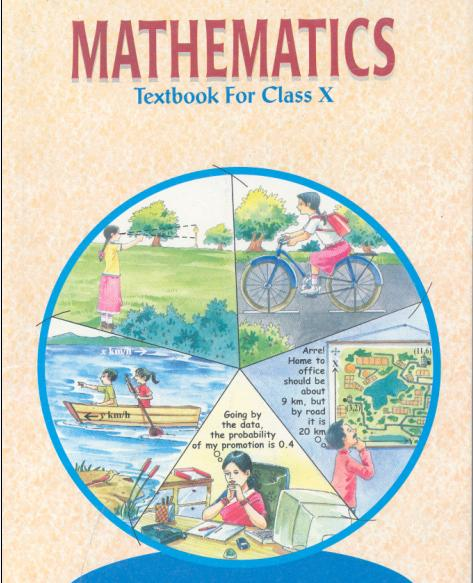## NCERT Solutions for Class 10 Maths Real Numbers

###### 1. Prove that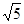is irrational.

Ans. Let us proveirrational by contradiction.

Let us suppose thatis rational. It means that we have co-prime integers a and b (b ≠ 0) such that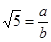b=a

Squaring both sides, we get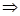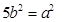(1)

It means that 5 is factor of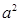Hence, 5 is also factor of a by Theorem. … (2)

If, 5 is factor of a, it means that we can write a = 5c for some integer c.

Substituting value of a in (1),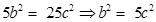It means that 5 is factor of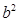.

Hence, 5 is also factor of b by Theorem. … (3)

From (2) and (3), we can say that 5 is factor of both a and b.

But, a and b are co-prime.

Therefore, our assumption was wrong.cannot be rational. Hence, it is irrational.

NCERT Solutions class-10 Maths Exercise 1.3

###### 2. Prove that (3 + 2) is irrational.

Ans. We will prove this by contradiction.

Let us suppose that (3+2) is rational.

It means that we have co-prime integers a and b (b ≠ 0) such that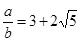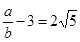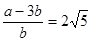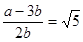(1)

a and b are integers.

It means L.H.S of (1) is rational but we know thatis irrational. It is not possible. Therefore, our supposition is wrong. (3+2) cannot be rational.

Hence, (3+2) is irrational.

NCERT Solutions class-10 Maths Exercise 1.3

###### 3. Prove that the following are irrationals.

(i)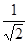(ii)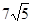(iii)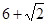Ans. (i) We can proveirrational by contradiction.

Let us suppose thatis rational.

It means we have some co-prime integers a and b (b ≠ 0) such that=ab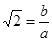(1)

R.H.S of (1) is rational but we know that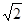is irrational.

It is not possible which means our supposition is wrong.

Therefore,cannot be rational.

Hence, it is irrational.

(ii) We can proveirrational by contradiction.

Let us suppose thatis rational.

It means we have some co-prime integers a and b (b ≠ 0) such that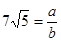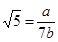(1)

R.H.S of (1) is rational but we know thatis irrational.

It is not possible which means our supposition is wrong.

Therefore,cannot be rational.

Hence, it is irrational.

(iii) We will proveirrational by contradiction.

Let us suppose that () is rational.

It means that we have co-prime integers a and b (b ≠ 0) such that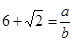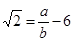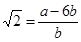(1)

a and b are integers.

It means L.H.S of (1) is rational but we know thatis irrational. It is not possible.

Therefore, our supposition is wrong. () cannot be rational.

Hence, () is irrational.

## NCERT Solutions class-10 Maths Exercise 1.3

NCERT Solutions Class 10 Maths PDF (Download) Free from myCBSEguide app and myCBSEguide website. Ncert solution class 10 Maths includes text book solutions from Mathematics Book. NCERT Solutions for CBSE Class 10 Maths have total 15 chapters. 10 Maths NCERT Solutions in PDF for free Download on our website. Ncert Maths class 10 solutions PDF and Maths ncert class 10 PDF solutions with latest modifications and as per the latest CBSE syllabus are only available in myCBSEguide.

## CBSE app for Class 10

To download NCERT Solutions for Class 10 Maths, Computer Science, Home Science,Hindi ,English, Social Science do check myCBSEguide app or website. myCBSEguide provides sample papers with solution, test papers for chapter-wise practice, NCERT solutions, NCERT Exemplar solutions, quick revision notes for ready reference, CBSE guess papers and CBSE important question papers. Sample Paper all are made available through the best app for CBSE students and myCBSEguide website.### 16 thoughts on “NCERT Solutions class-10 Maths Exercise 1.3”

1. Good job

2. It has a too short solution

Our tchr taught us in a step by step method to gain maximum marks

3. Nice

4. It is very goods for study and helpful also

5. Nice ????

6. Thanks for providing us this awesome and easiest formula
Thanks for once again ?

7. Nice?? i am very happy??

8. Nice????

9. It is a good way to understand this question.

10. Thank you for helping us

11. nice thanks it is very helpful for me.

12. Better, I can understand easily. Thanks

13. thanks for help me nice

14. Fantastic….. !!! Thanks a lot for helping a lot of students in such an easy manner….. keep it up…

15. Thanks for solving my problem

16. Nice I can understand nicely l like this